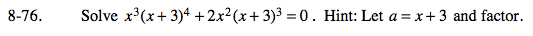### Home > PC > Chapter 8 > Lesson 8.2.1 > Problem8-76

8-76.Substitute a for x + 3:

Factor:

Set each factor = 0:

Substitute (x + 3) back in the expressions and solve.

x3a4 + 2x2a3 = 0

x2a3(ax + 2) = 0

x2a3 = 0 or ax + 2 = 0

x2(x + 3)3 = 0
x2 = 0
x = 0
(x + 3)3 = 0
x = −3

ax + 2 = 0
(x + 3)x + 2 = 0
x2 + 3x + 2 = 0
(x + 2)(x + 1) = 0
x = −2; x = −1